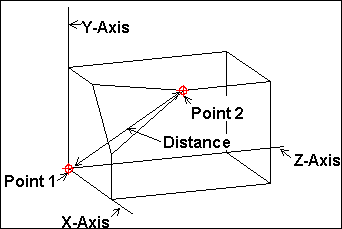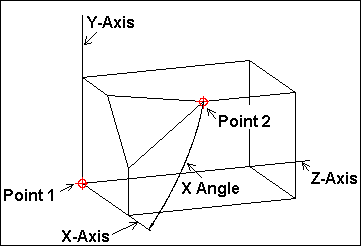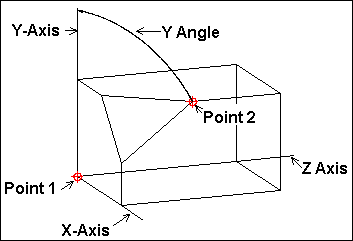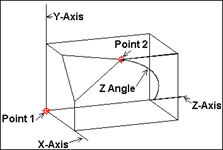# XYZ Coordinate System

When the XYZ Coordinate System is used, this command uses the location of the first point as the origin for the angular measurements.

Distance: The linear distance between the two points.X Angle: The angle from the X-Axis to the second point.Y Angle: The angle from the Y-Axis to the second point.Z Angle: The angle from the Z-Axis to the second point.